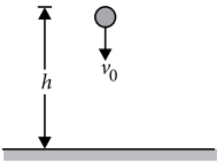Work done by Diff type of forces
Question

# A ball is thrown vertically downwards from a height of 20 m with an initial velocity ${\nu }_{0}$. It collides with the ground, loses 50 percent of its energy in collision and rebounds to the same height. The initial velocity ${\mathrm{\nu }}_{0}$ is (Take g =  )

Moderate
Solution

## The situation is shown in the figureLet $\nu$ be the velocity of the ball with which it collides with ground. Then according to the law of conservation of energy,Gain in kinetic energy = loss in potential energy Now, when the ball collides with the ground, 50% of its energy is lost and it rebounds to the same height h.$4gh-{v}_{0}^{2}=2gh$

Get Instant Solutions